Instance Selection

with Similarity-based, Evolutionary and Embedded MethodsMirosław Kordos

2009-2019

Purpose of Instance Selection

Good data preprocessing, including data selection is frequently the most important part of the prediction process, even more important than the optimal model parameters. This is because the models can be only as good as the data used for their learning. The list of data selection purposes begins with reduction of the data size and noise elimination, which both improve the data quality. They allow for achieving higher accuracy, shorter learning time and lower complexity of predictive models as well as easier data interpretation.

Data selection comprises feature selection and instance selection. Much more research has been done so far in feature selection. Since feature selection and instance selection are equally important. To bridge the gap my work focuses on instance selection and on the merge of instance and feature selection. However, as instance selection and feature selection are dependent on each other, in several places here feature selection is also considered, usually in connection with instance selection, but little new research in the feature selection is presented in my works.

Part 1. Instance Selection with Similarity-based Methods

Similarity-based instance selection methods can be divided into noise filters and condensation methods. In both cases they can be presented as a function

Weight(x) = F(Properties(neighbors(x)), Model(x, neighbors(x)))

where:

·      Weight(x) is a value that indicates the importance of the vector x (how much vector x is required or desired)

·      Properties of the neighbors of x can express local diversity of the data (e.g. standard deviation) or the trends in the data variability.

·      Model(x, neighbors(x)) is some predictor (classifier) that learns on neighbors(x) and predicts x; that can be k-NN, neural network, etc.

Then we set some threshold, and the instances with their weight below the threshold get rejected. But they still can be kept in the training dataset and their influence on the model can be multiplied by their weight. For example if the model is a neural network, then the error the network makes on each instance is multiplied by the instance weight, if the model is k-NN, then a weighed voting takes place.

In most of the instance selection methods, the Model(.) is based on some distance or neighborhood concept, as it is simple and computationally efficient. For example in the ENN algorithm (which is a noise filter),
k-NN is used to predict the class of each vector. It the real class of the vector is different than the predicted class then the vector is marked for removal, as it is considered to be noise. Finally all the marked vectors get removed. In case of instance weighting, the weight can be proportional to the percentage of the nearest neighbors that belong to the same class as the query instance.

In regression problems there are no classes, but that can be solved at least in two ways: by output attribute discretization and converting the regression problem into multi-label classification problem or by replacing the “same class” concept with some threshold distance between the real and the predicted value of each vector.

Condensation methods work by rejecting these vectors, which do not degrade the classification of their neighbors. But the concept used in weighing and regression problems can be applied in analogous way.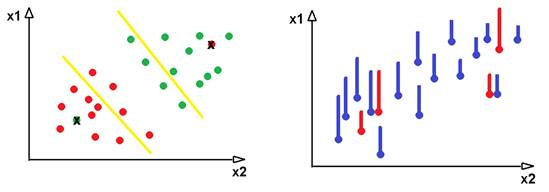Left:  only the correctly classified instances that are close to the decision boundary are required in classification (some exceptions apply). Right: instances very similar and very different from their neighbors can be removed in regression.

To illustrate the importance of properties of the neighborhood of a given point let us use a regression problem example. If the standard deviation of the neighbors of the point x is say 0.01 and the real value of the point differs from the predicted value by 0.5 – then probably this point makes noise and thus should be rejected. However, if the standard deviation is 10, then a difference of 0.5 looks OK.

This research area comprises various aspects of similarity-based instance selection, which were mentioned here, but particular instance selection algorithms, the use of clustering to improve instance selection speed and accuracy, ensemble methods in instance selection and the joined selection of features and instances.

My contribution in this area includes adaptation of the instance selection algorithms to regression problems, optimization of the Properties(Neighbors(x)) methods with weights reflecting the attribute, instance, distance, density and gradient properties, enhancements of ensemble methods in instance selection and concurrent instance and feature selection.

Part 2. Instance Selection with Evolutionary Methods

Evolutionary methods of instance selection optimize the following function:

Fitness(dataset) = F(accuracy, compression)

The first difference is that they optimize globally the whole training dataset, unlike the similarity-based methods, which deal with each instance separately. The optimization can be either single-objective or multi-objective, binary or real-value. In a single objective optimization we set an α parameter and optimize the function:

Fitness(dataset) = α accuracy+ (1- α) compression

We can also make the compression over features and instances in one optimization, then

compression = instanceCompression * featureCompression

Evolutionary or genetic algorithms use population of solutions, where each instance is encoded into one position in the chromosome and the typical genetic operators as fitness-based selection, crossover and mutation. However, the similarity-based methods can also use an equivalent of population – ensembles. The relation between ensembles and populations is also worth analyzing.

The second part is dedicated to various evolutionary methods of instance selection and instance weighting (in this case vectors are represented as real numbers between 0 and 1) and their properties and optimization as well as joining the similarity-based and evolutionary approach. It also deals with concurrent instance and feature selection. In that case the chromosome can consist of the instance part and the feature part, or two optimizations can be performed: one for instances and one for features and they can get synchronized at each iteration.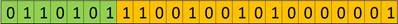Features (green) and instances (orange) encoded in the chromosome.

My contribution to that part includes solutions and improvements in speed and accuracy of instance weighting and selection by optimization of genetic and evolutionary algorithm process and its parameters. Different parameters best optimize the speed for CPU and for GPU calculations. Other ways to reduce the computational cost include the similarity-based methods and ensembles merged into in the evolutionary process. The evolutionary instance selection can be much less costly than the product of the number of fitness function evaluations by the cost of a single instance selection run, as much of the calculations can be simplified. For example, the full k-NN algorithm can be run only once at the beginning of optimization and the ordered distances can be intelligently cached.

It sometimes takes longer than similarity-based instance selection, but not always and the obtained results are much better, especially for classification tasks allowing for frequently much stronger compression than that of DROP3 or ENN+IB3 (which are considered one of the best similarity-based methods) and slightly higher prediction accuracy at the same time.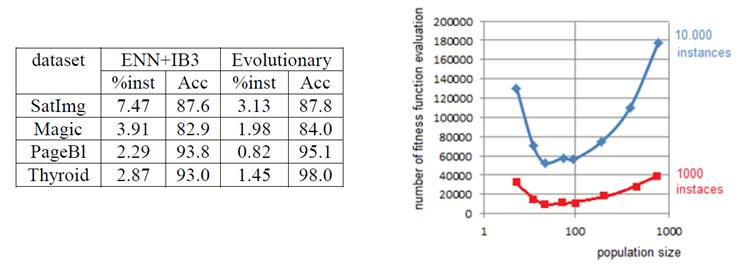Left: comparison of solution quality of instance selection methods. Right: population size optimization for CPU implementation (for GPU the optimal size can be much bigger and, unlike in CPU, it depends on the way fitness function is evaluated).

In multi-objective optimization the aim is to find the Pareto-front of non-dominated solutions, what corresponds to a simultaneous optimization for various α parameters. Recently we have obtained groundbreaking results with a modified version of NGSA-II algorithm applied to instance selection for regression problems. The method allows for obtaining a pool of solutions achieving good performance in terms of Pareto front of the minimization of rmse and maximization of compression and choosing the desired solution from the front. Different Pareto fronts can be obtained depending on several parameters of the instance selection process.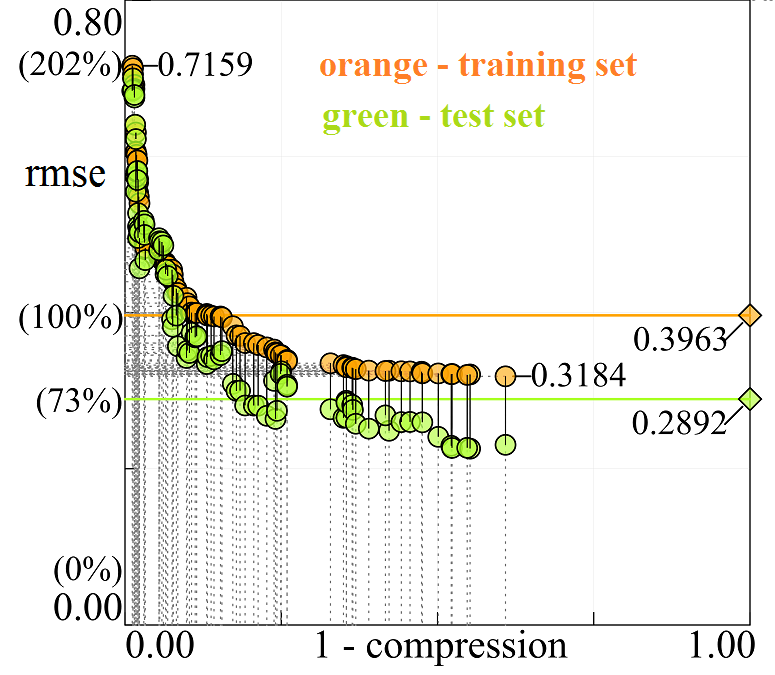Part 3. Instance Selection with Embedded Methods

The basic idea behind instance selection embedded into neural networks is that the instance properties are reflected by the error the trained network makes on that instance. If the error is very high then the instance can be considered noise and should be removed. However, it cannot be removed at the beginning of the network training, because at that time all vectors produce random errors, so the removal must be gradual and frequently better results can be obtained with instance weighting instead of removing. On contrary if the error is very low, the instance is probable situated far from decision boundary in the classification problem and also can be removed as it does not carry any useful information.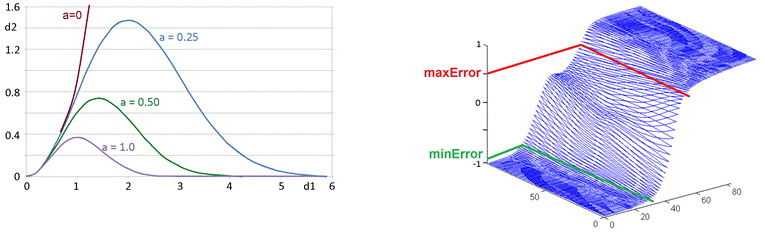Left:  bump functions are very efficient for instance weighting in regression.  Right: only the instances on the sigmoid slope matters in classification.

Different methods of instance detecting, weighting and removing have been developed, including merging the similarity-based methods with the neural network–based ones and joining instance selection with feature selection. Some of the methods required creating new network learning algorithms, as gradient-based method could not be applied. So also the learning algorithms developed by us will be presented. Next, the choice of particular noise removal method should be adjusted to the character of the data.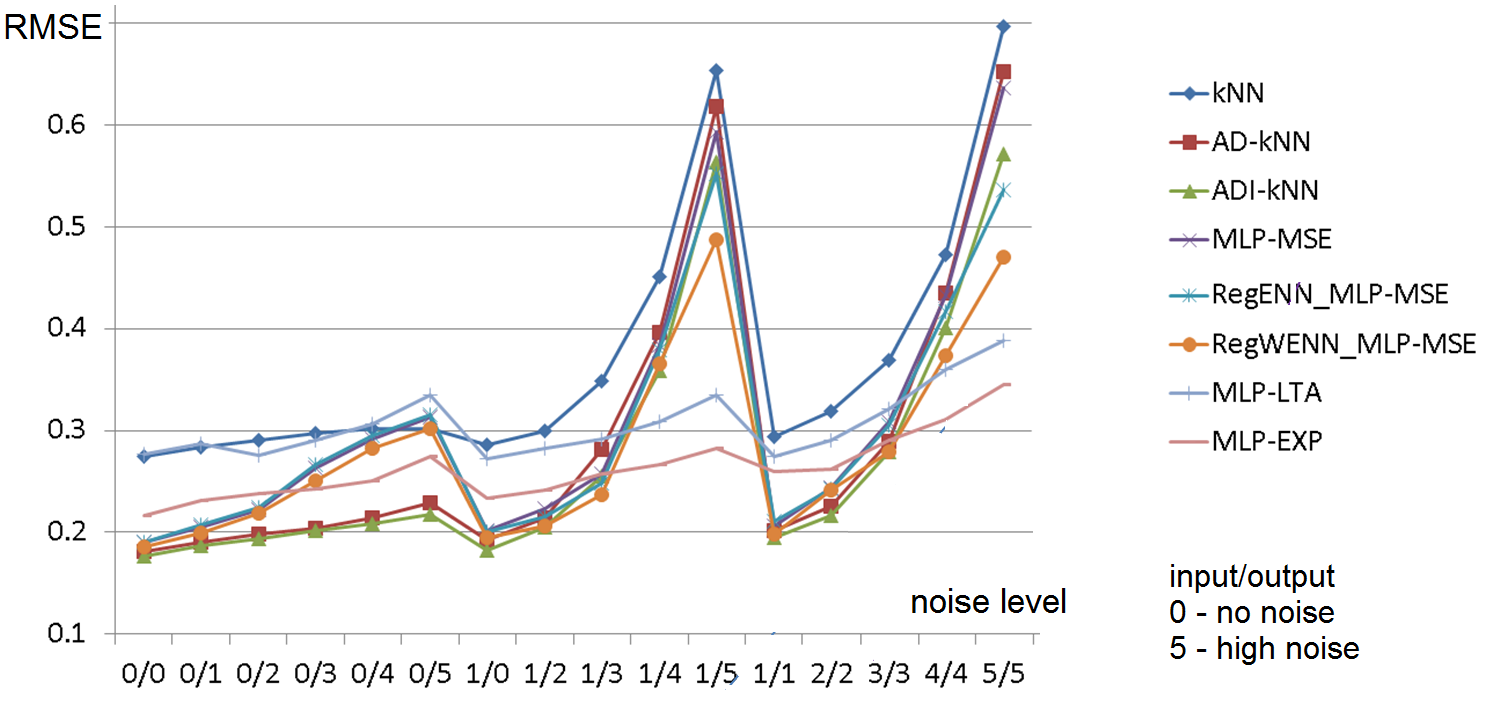How particular methods perform depending on the noise level in the training data.

Feature selection can be done by analysis of the weights in the trained network and then by removing these features, which propagates to the output week signals. Also feature selection can be joined with instance selection and this is also considered.

Feature selection in this context is closely related to decompositional rule extraction, as a similar analysis of signal propagation through the network weights is performed. The idea of logical rule extraction is to make the extracted rules enough simple to be understood by humans. This usually leads to some discretization of the input space in classification tasks. In regression tasks there are more ways to solve the problem.

There is also a connection between rule extraction and instance selection. Adjusting the network to rule extraction frequently also helps filtering out noisy instances and thus makes the network more noise-robust. The trade-off between the rule simplicity and the network accuracy can usually be addressed by incremental network structure, starting from the most simple and general areas, leading to the most accurate and complex one, so the user can make the analysis and any desired level of details.

Also here, as in similarity-based data selection, ensembles can be applied. Finally a sketch of how the embedded methods can be applied into other models will be presented.

Summary

The state-of-the-art instance selection methods and joined instance selection with feature selection are a very promising research area with practical applications. The solutions allow for improvements of the training data quality and as a consequence to improve the prediction process by allowing for higher accuracy and shorter training and prediction time and enable us to better understand the data properties and the properties of the processes represented by the data.

The software, datasets, detailed results of experiments, papers, links to related resources, and other information are successively being placed on my web page.